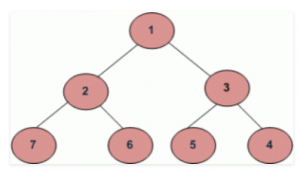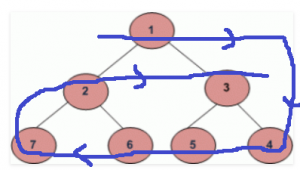Related Articles

# Clockwise Spiral Traversal of Binary Tree

• Difficulty Level : Medium
• Last Updated : 24 Oct, 2019

Given a Binary Tree. The task is to print the circular clockwise spiral order traversal of the given binary tree.For the above binary tree, the circular clockwise spiral order traversal will be 1, 4, 5, 6, 7, 2, 3.Examples:

```Input :
10
/     \
12       13
/     \
14       15
/   \     /  \
21   22   23   24
Output : 10, 24, 23, 22, 21, 12, 13, 15, 14
```

## Recommended: Please try your approach on {IDE} first, before moving on to the solution.

Approach:

1. First calculate the width of the given tree.
2. Create an auxiliary 2D array of order (width*width)
3. Do level order traversal of the binary tree and store levels in the newly created 2D matrix one by one in respective rows. That is, store nodes at level 0 at row indexed 0, nodes at level 1 at row indexed 1 and so on.
4. Finally, traverse the 2d array in the below fashion:
• Start from the first row from left to right and print elements.
• Then traverse the last row from right to left and print elements.
• Again traverse the second row from left to right and print.
• Then second last row from right to left and so on and repeat the steps until the complete 2-D array is traversed.

Below is the implementation of the above approach:

## C++

 `// C++ program for Clockwise Spiral Traversal ``// of Binary Tree `` ` `#include  ``using` `namespace` `std; `` ` `// A Tree node ``struct` `Node { ``    ``int` `key; ``    ``struct` `Node *left, *right; ``}; `` ` `// Utility function to create a new node ``Node* newNode(``int` `key) ``{ ``    ``Node* temp = ``new` `Node; ``    ``temp->key = key; ``    ``temp->left = temp->right = NULL; ``    ``return` `(temp); ``} ``//function to calculate the height of the tree``int` `findHeight(``struct` `Node* node)``{``    ``//Base condition``    ``if``(node == NULL) ``return` `0;``    ``int` `leftHeight = findHeight(node->left);``    ``int` `rightHeight = findHeight(node->right);``    ``//return maximum of left or right subtree height addition with one``    ``return` `1+(leftHeight > rightHeight ? leftHeight : rightHeight );``}``// Function to find the width of tree ``void` `findWidth(``struct` `Node* node, ``int``& maxValue, ``            ``int``& minValue, ``int` `hd) ``{ ``    ``if` `(node == NULL) ``        ``return``; `` ` `    ``if` `(hd > maxValue) { ``        ``maxValue = hd; ``    ``} `` ` `    ``if` `(hd < minValue) { ``        ``minValue = hd; ``    ``} `` ` `    ``findWidth(node->left, maxValue, minValue, hd - 1); ``    ``findWidth(node->right, maxValue, minValue, hd + 1); ``} `` ` `// Function to traverse the tree and ``// store level order traversal in a matrix ``void` `BFS(``int``** mtrx, ``struct` `Node* node) ``{ ``    ``// Create queue for storing ``    ``// the addresses of nodes ``    ``queue<``struct` `Node*> qu; `` ` `    ``qu.push(node); `` ` `    ``int` `i = -1, j = -1; `` ` `    ``struct` `Node* poped_node = NULL; `` ` `    ``while` `(!qu.empty()) { `` ` `        ``i++; `` ` `        ``int` `qsize = qu.size(); `` ` `        ``while` `(qsize--) { ``            ``j++; `` ` `            ``poped_node = qu.front(); `` ` `            ``// Store data of node into the matrix ``            ``mtrx[i][j] = poped_node->key; ``            ``qu.pop(); `` ` `            ``if` `(poped_node->left != NULL) { ``                ``qu.push(poped_node->left); ``            ``} `` ` `            ``if` `(poped_node->right != NULL) { ``                ``qu.push(poped_node->right); ``            ``} ``        ``} `` ` `        ``j = -1; ``    ``} ``} `` ` `// Function for Clockwise Spiral Traversal ``// of Binary Tree ``void` `traverse_matrix(``int``** mtrx, ``int` `height, ``int` `width) ``{ ``    ``int` `j = 0, k1 = 0, k2 = 0, k3 = height - 1; ``    ``int` `k4 = width - 1; `` ` `    ``for` `(``int` `round = 0; round < height / 2; round++) { ``        ``for` `(j = k2; j < width; j++) { `` ` `            ``// only print those values which ``            ``// are not MAX_INTEGER ``            ``if` `(mtrx[k1][j] != INT_MAX) { ``                ``cout << mtrx[k1][j] << ``", "``; ``            ``} ``        ``} `` ` `        ``k2 = 0; ``        ``k1++; `` ` `        ``for` `(j = k4; j >= 0; j--) { `` ` `            ``// only print those values which are ``            ``// not MAX_INTEGER ``            ``if` `(mtrx[k3][j] != INT_MAX) { ``                ``cout << mtrx[k3][j] << ``", "``; ``            ``} ``        ``} `` ` `        ``k4 = width - 1; ``        ``k3--; ``    ``} `` ` `    ``// condition (one row may be left traversing) ``    ``// if number of rows in matrix are odd ``    ``if` `(height % 2 != 0) { ``        ``for` `(j = k2; j < width; j++) { `` ` `            ``// only print those values which are ``            ``// not MAX_INTEGER ``            ``if` `(mtrx[k1][j] != INT_MAX) { ``                ``cout << mtrx[k1][j] << ``", "``; ``            ``} ``        ``} ``    ``} ``} `` ` `// A utility function to print clockwise ``// spiral traversal of tree ``void` `printPattern(``struct` `Node* node) ``{ ``    ``// max, min has taken for ``    ``// calculating width of tree ``    ``int` `max_value = INT_MIN; ``    ``int` `min_value = INT_MAX; ``    ``int` `hd = 0; `` ` `    ``// calculate the width of a tree ``    ``findWidth(node, max_value, min_value, hd); ``    ``int` `width = max_value + ``abs``(min_value); ``    ` `    ``//calculate the height of the tree``    ``int` `height = findHeight(node); ``    ` `    ``// use double pointer to create 2D array ``    ``int``** mtrx = ``new` `int``*[height]; `` ` `    ``// initialize width for each row of matrix ``    ``for` `(``int` `i = 0; i < height; i++) { ``        ``mtrx[i] = ``new` `int``[width]; ``    ``} `` ` `    ``// initialize complete matrix with ``    ``// MAX INTEGER(purpose garbage) ``    ``for` `(``int` `i = 0; i < height; i++) { ``        ``for` `(``int` `j = 0; j < width; j++) { ``            ``mtrx[i][j] = INT_MAX; ``        ``} ``    ``} `` ` `    ``// Store the BFS traversal of the tree ``    ``// into the 2-D matrix ``    ``BFS(mtrx, node); `` ` `    ``// Print the circular clockwise spiral ``    ``// traversal of the tree ``    ``traverse_matrix(mtrx, height, width); `` ` `    ``// release extra memory ``    ``// allocated for matrix ``    ``free``(mtrx); ``} `` ` `// Driver Code ``int` `main() ``{ ``    ``/*     10 ``        ``/     \ ``    ``12     13 ``        ``/     \ ``        ``14     15 ``        ``/ \     / \ ``        ``21 22 23 24 ``         ` `    ``Let us create Binary Tree as shown ``    ``in above example */`` ` `    ``Node* root = newNode(10); ``    ``root->left = newNode(12); ``    ``root->right = newNode(13); ``   ` `    ``root->right->left = newNode(14); ``    ``root->right->right = newNode(15); ``   ` `    ``root->right->left->left = newNode(21); ``    ``root->right->left->right = newNode(22); ``    ``root->right->right->left = newNode(23); ``    ``root->right->right->right = newNode(24); `` ` `    ``cout << ``"Circular Clockwise Spiral Traversal : \n"``; `` ` `    ``printPattern(root); `` ` `    ``return` `0; ``} ``// This code is contributed by MOHAMMAD MUDASSIR`

## Python3

 `# Python3 program for Clockwise Spiral ``# Traversal of Binary Tree``INT_MAX ``=` `2``*``*``31``INT_MIN ``=` `-``2``*``*``31`` ` `# Binary tree node ``class` `newNode: `` ` `    ``# Constructor to create a newNode ``    ``def` `__init__(``self``, data): ``        ``self``.key ``=` `data ``        ``self``.left ``=` `None``        ``self``.right ``=` `None``         ` `# Function to find the width of tree ``def` `findWidth(node, maxValue, minValue, hd):`` ` `    ``if` `(node ``=``=` `None``):``        ``return`` ` `    ``if` `(hd > maxValue[``0``]):``        ``maxValue[``0``] ``=` `hd ``     ` `    ``if` `(hd < minValue[``0``]):``        ``minValue[``0``] ``=` `hd `` ` `    ``findWidth(node.left, maxValue,``                         ``minValue, hd ``-` `1``) ``    ``findWidth(node.right, maxValue,``                          ``minValue, hd ``+` `1``) `` ` `# Function to traverse the tree and ``# store level order traversal in a matrix ``def` `BFS(mtrx,node): `` ` `    ``# Create queue for storing ``    ``# the addresses of nodes ``    ``qu ``=` `[] `` ` `    ``qu.append(node) `` ` `    ``i ``=` `-``1``    ``j ``=` `-``1`` ` `    ``poped_node ``=` `None`` ` `    ``while` `(``len``(qu)):``        ``i ``+``=` `1`` ` `        ``qsize ``=` `len``(qu)`` ` `        ``while` `(qsize > ``0``):``            ``qsize ``-``=` `1``            ``j ``+``=` `1`` ` `            ``poped_node ``=` `qu[``0``] `` ` `            ``# Store data of node into the matrix ``            ``mtrx[i][j] ``=` `poped_node.key ``            ``qu.pop(``0``) `` ` `            ``if` `(poped_node.left !``=` `None``):``                ``qu.append(poped_node.left) ``             ` `            ``if` `(poped_node.right !``=` `None``): ``                ``qu.append(poped_node.right) ``             ` `        ``j ``=` `-``1``     ` `# Function for Clockwise Spiral ``# Traversal of Binary Tree ``def` `traverse_matrix(mtrx, width):`` ` `    ``j ``=` `0``    ``k1 ``=` `0``    ``k2 ``=` `0``    ``k3 ``=` `width ``-` `1``    ``k4 ``=` `width ``-` `1`` ` `    ``for` `round` `in` `range``(width ``/``/` `2``): ``        ``for` `j ``in` `range``(k2, width): `` ` `            ``# only prthose values which ``            ``# are not MAX_INTEGER ``            ``if` `(mtrx[k1][j] !``=` `INT_MAX):``                ``print``(mtrx[k1][j], ``", "``, end ``=` `"")``        ``k2 ``=` `0``        ``k1 ``+``=` `1`` ` `        ``for` `j ``in` `range``(k4, ``-``1``, ``-``1``):`` ` `            ``# only prthose values which are ``            ``# not MAX_INTEGER ``            ``if` `(mtrx[k3][j] !``=` `INT_MAX):``                ``print``(mtrx[k3][j], ``", "``, end ``=` `"") ``         ` `        ``k4 ``=` `width ``-` `1``        ``k3 ``-``=` `1`` ` `    ``# condition (one row may be left traversing) ``    ``# if number of rows in matrix are odd ``    ``if` `(width ``%` `2` `!``=` `0``): ``        ``for` `j ``in` `ramge(k2, width):`` ` `            ``# only prthose values which ``            ``# are not MAX_INTEGER ``            ``if` `(mtrx[k1][j] !``=` `INT_MAX):``                ``print``(mtrx[k1][j], ``", "``, end ``=` `"")``                 ` `# A utility function to prclockwise ``# spiral traversal of tree ``def` `printPattern(node): `` ` `    ``# max, min has taken for ``    ``# calculating width of tree ``    ``max_value ``=` `[INT_MIN] ``    ``min_value ``=` `[INT_MAX ]``    ``hd ``=` `0`` ` `    ``# calculate the width of a tree ``    ``findWidth(node, max_value, min_value, hd) ``    ``width ``=` `max_value[``0``] ``+` `abs``(min_value[``0``]) `` ` `    ``# use double pointer to ``    ``# create 2D array ``    ``mtrx ``=` `[``0``]``*``width `` ` `    ``# initialize width for each``    ``# row of matrix ``    ``for` `i ``in` `range``(width): ``        ``mtrx[i] ``=` `[``0``] ``*` `width``     ` `    ``# initialize complete matrix with ``    ``# MAX INTEGER(purpose garbage) ``    ``for` `i ``in` `range``(width): ``        ``for` `j ``in` `range``(width): ``            ``mtrx[i][j] ``=` `INT_MAX ``         ` `    ``# Store the BFS traversal of the ``    ``# tree into the 2-D matrix ``    ``BFS(mtrx, node)``     ` `    ``# Print the circular clockwise spiral ``    ``# traversal of the tree ``    ``traverse_matrix(mtrx, width) ``         ` `# Driver Code``if` `__name__ ``=``=` `'__main__'``:``     ` `    ``"""     10 ``        ``/     \ ``    ``12     13 ``        ``/     \ ``        ``14     15 ``        ``/ \     / \ ``        ``21 22 23 24 ``         ` `    ``Let us create Binary Tree as shown ``    ``in above example """`` ` `    ``root ``=` `newNode(``10``) ``    ``root.left ``=` `newNode(``12``) ``    ``root.right ``=` `newNode(``13``) `` ` `    ``root.right.left ``=` `newNode(``14``) ``    ``root.right.right ``=` `newNode(``15``) `` ` `    ``root.right.left.left ``=` `newNode(``21``) ``    ``root.right.left.right ``=` `newNode(``22``) ``    ``root.right.right.left ``=` `newNode(``23``) ``    ``root.right.right.right ``=` `newNode(``24``) `` ` `    ``print``(``"Circular Clockwise Spiral Traversal :"``) `` ` `    ``printPattern(root)`` ` `# This code is contributed by ``# SHUBHAMSINGH10`
Output:
```Circular Clockwise Spiral Traversal :
10, 24, 23, 22, 21, 12, 13, 15, 14,
```

Attention reader! Don’t stop learning now. Get hold of all the important DSA concepts with the DSA Self Paced Course at a student-friendly price and become industry ready.  To complete your preparation from learning a language to DS Algo and many more,  please refer Complete Interview Preparation Course.

In case you wish to attend live classes with experts, please refer DSA Live Classes for Working Professionals and Competitive Programming Live for Students.

My Personal Notes arrow_drop_up